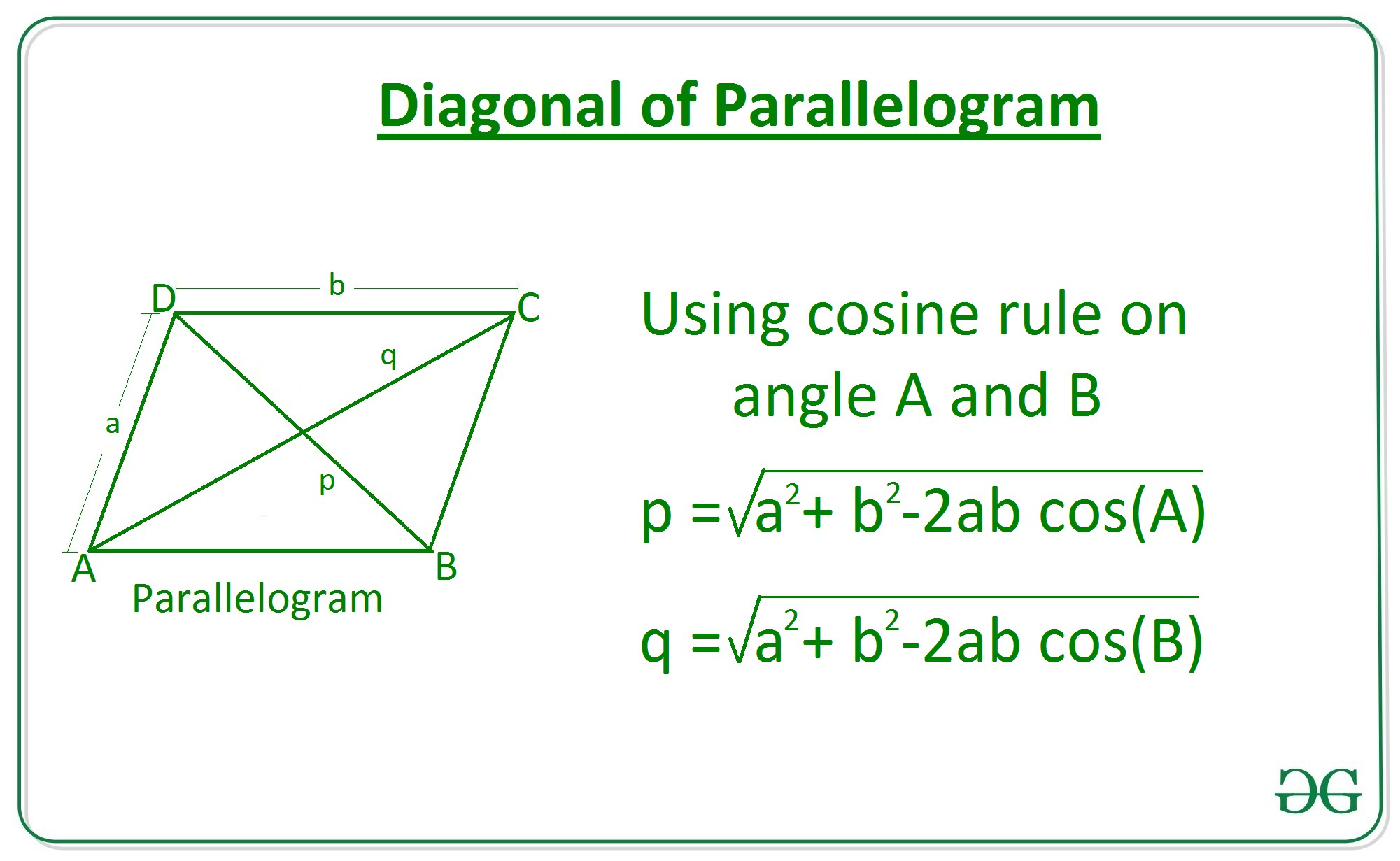Related Articles

# Length of diagonal of a parallelogram using adjacent sides and angle between them

• Last Updated : 08 Apr, 2021

Given two integers a and b where a and b represents the length of adjacent sides of a parallelogram and an angle 0 between them, the task is to find the length of diagonal of the parallelogram.

Examples:

Attention reader! All those who say programming isn't for kids, just haven't met the right mentors yet. Join the  Demo Class for First Step to Coding Coursespecifically designed for students of class 8 to 12.

The students will get to learn more about the world of programming in these free classes which will definitely help them in making a wise career choice in the future.

Input: a = 6, b = 10, 0=30
Output: 6.14

Input: a = 3, b = 5, 0=45
Output: 3.58

Approach: Consider a parallelogram ABCD with sides a and b, now apply cosine rule at angle A in the triangle ABD to find the length of diagonal p, similarly find diagonal q from triangle ABC.Therefore the diagonals is given by:## C++

 // C++ program to find length// Of diagonal of a parallelogram// Using sides and angle between them.#include using namespace std;#define PI 3.147 // Function to return the length// Of diagonal of a parallelogram// using sides and angle between them.double Length_Diagonal(int a, int b, double theta){    double diagonal = sqrt((pow(a, 2) + pow(b, 2)) -                      2 * a * b * cos(theta * (PI / 180)));     return diagonal;} // Driver Codeint main(){     // Given sides    int a = 3;    int b = 5;     // Given angle    double theta = 45;     // Function call    double ans = Length_Diagonal(a, b, theta);     // Print the final answer    printf("%.2f", ans);} // This code is contributed by Amit Katiyar

## Java

 // Java program to find length// Of diagonal of a parallelogram// Using sides and angle between them.class GFG{ // Function to return the length// Of diagonal of a parallelogram// using sides and angle between them.static double Length_Diagonal(int a, int b,                              double theta){    double diagonal = Math.sqrt((Math.pow(a, 2) +                                 Math.pow(b, 2)) -                                 2 * a * b *                                 Math.cos(theta *                                 (Math.PI / 180)));     return diagonal;} // Driver Codepublic static void main(String[] args){         // Given sides    int a = 3;    int b = 5;     // Given angle    double theta = 45;     // Function call    double ans = Length_Diagonal(a, b, theta);     // Print the final answer    System.out.printf("%.2f", ans);}} // This code is contributed by amal kumar choubey

## Python3

 # Python3 Program to find length# Of diagonal of a parallelogram# Using sides and angle between them. import math    # Function to return the length# Of diagonal of a parallelogram# using sides and angle between them. def Length_Diagonal(a, b, theta):        diagonal = math.sqrt( ((a**2) + (b**2))    - 2 * a*b * math.cos(math.radians(theta)))         return diagonal    # Driver Code # Given Sidesa = 3b = 5 # Given Angletheta = 45   # Function Call ans = Length_Diagonal(a, b, theta)    # Print the final answerprint(round(ans, 2))

## C#

 // C# program to find length// Of diagonal of a parallelogram// Using sides and angle between them.using System; class GFG{ // Function to return the length// Of diagonal of a parallelogram// using sides and angle between them.static double Length_Diagonal(int a, int b,                              double theta){    double diagonal = Math.Sqrt((Math.Pow(a, 2) +                                 Math.Pow(b, 2)) -                                 2 * a * b *                                 Math.Cos(theta *                                (Math.PI / 180)));     return diagonal;} // Driver Codepublic static void Main(String[] args){         // Given sides    int a = 3;    int b = 5;     // Given angle    double theta = 45;     // Function call    double ans = Length_Diagonal(a, b, theta);     // Print the readonly answer    Console.Write("{0:F2}", ans);}} // This code is contributed by amal kumar choubey

## Javascript

 
Output:
3.58

Time Complexity: O(1)
Auxiliary Space: O(1)

My Personal Notes arrow_drop_up